# Install mpi4py on Windows 10

#### Install Microsoft MPI

You need to run both files msmpisdk.msi and MSMpiSetup.exe

#### Add $PATH$ in the Environment Variables.

$PATH$ is where you install the Microsoft MPI. In my case:

C:\Program Files (x86)\Microsoft SDKs\MPI

#### Install package in Anaconda

conda install mpi4py

#### And finally, test the installation

from mpi4py import MPI
comm = MPI.COMM_WORLD
rank = comm.Get_rank()
print (“hello world from process “, rank)

# DL libraries

1. Install Keras on Anaconda Windows

• Install TDM GCC x64. Add PATH
• Install Anaconda x64.
• Open the Anaconda prompt
• Run conda update conda
• Run conda update --all
• Run conda install mingw libpython
• Install the latest version of Theano, pip install git+git://github.com/Theano/Theano.git
• Run pip install git+git://github.com/fchollet/keras.git

2. Install pyCUDA

• Get the the PyCUDA prebuilt binary from Christoph Golke (his page is an invaluable resource for installing Python packages on Windows).
• Select the right combination of OS (for me it was pycuda-2016.1.2+cuda8044-cp35-cp35m-win_amd64.whl)
• Use pip to install the above package.  Simply type this in your Anaconda prompt
pip install <directory>\pycuda-2016.1.2+cuda8044-cp35-cp35m-win_amd64.whl

# Beginners Guide to Topic Modeling in Python

## Introduction

Analytics Industry is all about obtaining the “Information” from the data. With the growing amount of data in recent years, that too mostly unstructured, it’s difficult to obtain the relevant and desired information. But, technology has developed some powerful methods which can be used to mine through the data and fetch the information that we are looking for.

One such technique in the field of text mining is Topic Modelling. As the name suggests, it is a process to automatically identify topics present in a text object and to derive hidden patterns exhibited by a text corpus. Thus, assisting better decision making.

Topic Modelling is different from rule-based text mining approaches that use regular expressions or dictionary based keyword searching techniques. It is an unsupervised approach used for finding and observing the bunch of words (called “topics”) in large clusters of texts.

Topics can be defined as “a repeating pattern of co-occurring terms in a corpus”. A good topic model should result in – “health”, “doctor”, “patient”, “hospital” for a topic – Healthcare, and “farm”, “crops”, “wheat” for a topic – “Farming”.

Topic Models are very useful for the purpose for document clustering, organizing large blocks of textual data, information retrieval from unstructured text and feature selection. For Example – New York Times are using topic models to boost their user – article recommendation engines. Various professionals are using topic models for recruitment industries where they aim to extract latent features of job descriptions and map them to right candidates. They are being used to organize large datasets of emails, customer reviews, and user social media profiles.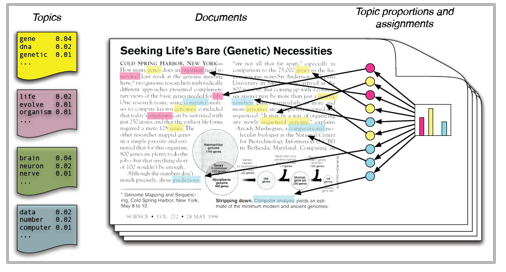So, if you aren’t sure about the complete process of topic modeling, this guide would introduce you to various concepts followed by its implementation in python.

## Table of Content

• Latent Dirichlet Allocation for Topic Modeling
• Parameters of LDA
• Python Implementation
• Preparing documents
• Cleaning and Preprocessing
• Preparing document term matrix
• Running LDA model
• Results
• Tips to improve results of topic modelling
• Frequency Filter
• Part of Speech Tag Filter
• Batch Wise LDA
• Topic Modeling for Feature Selection

## Latent Dirichlet Allocation for Topic Modeling

There are many approaches for obtaining topics from a text such as – Term Frequency and Inverse Document Frequency. NonNegative Matrix Factorization techniques. Latent Dirichlet Allocation is the most popular topic modeling technique and in this article, we will discuss the same.

LDA assumes documents are produced from a mixture of topics. Those topics then generate words based on their probability distribution. Given a dataset of documents, LDA backtracks and tries to figure out what topics would create those documents in the first place.

LDA is a matrix factorization technique. In vector space, any corpus (collection of documents) can be represented as a document-term matrix. The following matrix shows a corpus of N documents D1, D2, D3 … Dn and vocabulary size of M words W1,W2 .. Wn. The value of i,j cell gives the frequency count of word Wj in Document Di.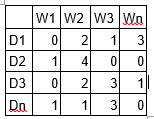LDA converts this Document-Term Matrix into two lower dimensional matrices – M1 and M2.
M1 is a document-topics matrix and M2 is a topic – terms matrix with dimensions (N,  K) and (K, M) respectively, where N is the number of documents, K is the number of topics and M is the vocabulary size.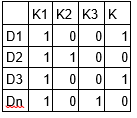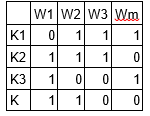Notice that these two matrices already provides topic word and document topic distributions, However, these distribution needs to be improved, which is the main aim of LDA. LDA makes use of sampling techniques in order to improve these matrices.

It Iterates through each word “w” for each document “d” and tries to adjust the current topic – word assignment with a new assignment. A new topic “k” is assigned to word “w” with a probability P which is a product of two probabilities p1 and p2.

For every topic, two probabilities p1 and p2 are calculated. P1 – p(topic t / document d) = the proportion of words in document d that are currently assigned to topic t. P2 – p(word w / topic t) = the proportion of assignments to topic t over all documents that come from this word w.

The current topic – word assignment is updated with a new topic with the probability, product of p1 and p2 . In this step, the model assumes that all the existing word – topic assignments except the current word are correct. This is essentially the probability that topic t generated word w, so it makes sense to adjust the current word’s topic with new probability.

After a number of iterations, a steady state is achieved where the document topic and topic term distributions are fairly good. This is the convergence point of LDA.

### Parameters of LDA

Alpha and Beta Hyperparameters – alpha represents document-topic density and Beta represents topic-word density. Higher the value of alpha, documents are composed of more topics and lower the value of alpha, documents contain fewer topics. On the other hand, higher the beta, topics are composed of a large number of words in the corpus, and with the lower value of beta, they are composed of few words.

Number of Topics – Number of topics to be extracted from the corpus. Researchers have developed approaches to obtain an optimal number of topics by using Kullback Leibler Divergence Score. I will not discuss this in detail, as it is too mathematical. For understanding, one can refer to this original paper on the use of KL divergence.

Number of Topic Terms – Number of terms composed in a single topic. It is generally decided according to the requirement. If the problem statement talks about extracting themes or concepts, it is recommended to choose a higher number, if problem statement talks about extracting features or terms, a low number is recommended.

Number of Iterations / passes – Maximum number of iterations allowed to LDA algorithm for convergence.

## Running in python

### Preparing Documents

Here are the sample documents combining together to form a corpus.

doc1 = "Sugar is bad to consume. My sister likes to have sugar, but not my father."
 doc2 = "My father spends a lot of time driving my sister around to dance practice."
 doc3 = "Doctors suggest that driving may cause increased stress and blood pressure."
 doc4 = "Sometimes I feel pressure to perform well at school, but my father never seems to drive my sister to do better."
 doc5 = "Health experts say that Sugar is not good for your lifestyle."

 # compile documents
 doc_complete = [doc1, doc2, doc3, doc4, doc5]

### Cleaning and Preprocessing

Cleaning is an important step before any text mining task, in this step, we will remove the punctuations, stopwords and normalize the corpus.

 
 from nltk.corpus import stopwords 
 from nltk.stem.wordnet import WordNetLemmatizer
 import string
stop = set(stopwords.words('english'))
 exclude = set(string.punctuation) 
 lemma = WordNetLemmatizer()
def clean(doc):
     stop_free = " ".join([i for i in doc.lower().split() if i not in stop])
     punc_free = ''.join(ch for ch in stop_free if ch not in exclude)
     normalized = " ".join(lemma.lemmatize(word) for word in punc_free.split())
     return normalized

doc_clean = [clean(doc).split() for doc in doc_complete]        
 

### Preparing Document-Term Matrix

All the text documents combined is known as the corpus. To run any mathematical model on text corpus, it is a good practice to convert it into a matrix representation. LDA model looks for repeating term patterns in the entire DT matrix. Python provides many great libraries for text mining practices, “gensim” is one such clean and beautiful library to handle text data. It is scalable, robust and efficient. Following code shows how to convert a corpus into a document-term matrix.

 
# Importing Gensim import gensim from gensim import corpora  # Creating the term dictionary of our courpus, where every unique term is assigned an index. dictionary = corpora.Dictionary(doc_clean)
 # Converting list of documents (corpus) into Document Term Matrix using dictionary prepared above. doc_term_matrix = [dictionary.doc2bow(doc) for doc in doc_clean]
 

### Running LDA Model

Next step is to create an object for LDA model and train it on Document-Term matrix. The training also requires few parameters as input which are explained in the above section. The gensim module allows both LDA model estimation from a training corpus and inference of topic distribution on new, unseen documents.

 
# Creating the object for LDA model using gensim library Lda = gensim.models.ldamodel.LdaModel
 # Running and Trainign LDA model on the document term matrix. ldamodel = Lda(doc_term_matrix, num_topics=3, id2word = dictionary, passes=50)

 

### Results


 print(ldamodel.print_topics(num_topics=3, num_words=3))
 ['0.168*health + 0.083*sugar + 0.072*bad, '0.061*consume + 0.050*drive + 0.050*sister, '0.049*pressur + 0.049*father + 0.049*sister]
 

Each line is a topic with individual topic terms and weights. Topic1 can be termed as Bad Health, and Topic3 can be termed as Family.

## Tips to improve results of topic modeling

The results of topic models are completely dependent on the features (terms) present in the corpus. The corpus is represented as document term matrix, which in general is very sparse in nature. Reducing the dimensionality of the matrix can improve the results of topic modelling. Based on my practical experience, there are few approaches which do the trick.

1. Frequency Filter – Arrange every term according to its frequency. Terms with higher frequencies are more likely to appear in the results as compared ones with low frequency. The low frequency terms are essentially weak features of the corpus, hence it is a good practice to get rid of all those weak features. An exploratory analysis of terms and their frequency can help to decide what frequency value should be considered as the threshold.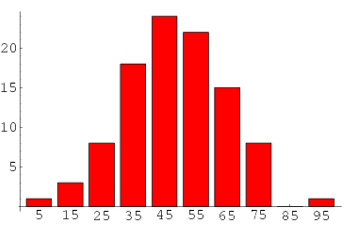2. Part of Speech Tag Filter – POS tag filter is more about the context of the features than frequencies of features. Topic Modelling tries to map out the recurring patterns of terms into topics. However, every term might not be equally important contextually. For example, POS tag IN contain terms such as – “within”, “upon”, “except”. “CD” contains – “one”,”two”, “hundred” etc. “MD” contains “may”, “must” etc. These terms are the supporting words of a language and can be removed by studying their post tags.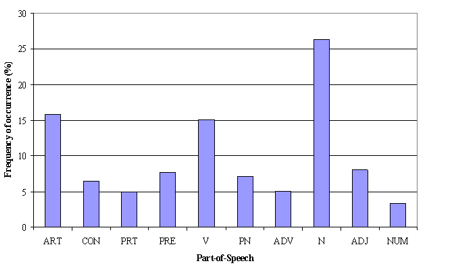3. Batch Wise LDA –
In order to retrieve most important topic terms, a corpus can be divided into batches of fixed sizes. Running LDA multiple times on these batches will provide different results, however, the best topic terms will be the intersection of all batches.

## Topic Modelling for Feature Selection

Sometimes LDA can also be used as feature selection technique. Take an example of text classification problem where the training data contain category wise documents. If LDA is running on sets of category wise documents. Followed by removing common topic terms across the results of different categories will give the best features for a category.

## Endnotes

With this, we come to this end of tutorial on Topic Modeling. I hope this will help you to improve your knowledge to work on text data. To reap maximum benefits out of this tutorial, I’d suggest you practice the codes side by side and check the results.

# What is LDA?

Latent Dirichlet allocation (LDA) is a topic model that generates topics based on word frequency from a set of documents. LDA is particularly useful for finding reasonably accurate mixtures of topics within a given document set.

# LDA walkthrough

This walkthrough goes through the process of generating an LDA model with a highly simplified document set. This is not an exhaustive explanation of LDA. The goal of this walkthrough is to guide users through key steps in preparing their data and providing example output.

## Packages required

This walkthrough uses the following Python packages:

• NLTK, a natural language toolkit for Python. A useful package for any natural language processing.
• For Mac/Unix with pip: $sudo pip install -U nltk. • stop_words, a Python package containing stop words. • For Mac/Unix with pip: $ sudo pip install stop-words.
• gensim, a topic modeling package containing our LDA model.
• For Mac/Unix with pip: $sudo pip install gensim. ## Importing your documents Here is our sample documents: doc_a = "Brocolli is good to eat. My brother likes to eat good brocolli, but not my mother." doc_b = "My mother spends a lot of time driving my brother around to baseball practice." doc_c = "Some health experts suggest that driving may cause increased tension and blood pressure." doc_d = "I often feel pressure to perform well at school, but my mother never seems to drive my brother to do better." doc_e = "Health professionals say that brocolli is good for your health." # compile sample documents into a list doc_set = [doc_a, doc_b, doc_c, doc_d, doc_e] ## Cleaning your documents Data cleaning is absolutely crucial for generating a useful topic model: as the saying goes, “garbage in, garbage out.” The steps below are common to most natural language processing methods: • Tokenizing: converting a document to its atomic elements. • Stopping: removing meaningless words. • Stemming: merging words that are equivalent in meaning. ### Tokenization Tokenization segments a document into its atomic elements. In this case, we are interested in tokenizing to words. Tokenization can be performed many ways–we are using NLTK’s tokenize.regexp module: from nltk.tokenize import RegexpTokenizer tokenizer = RegexpTokenizer(r'\w+') The above code will match any word characters until it reaches a non-word character, like a space. This is a simple solution, but can cause problems for words like “don’t” which will be read as two tokens, “don” and “t.” NLTK provides a number of pre-constructed tokenizers like nltk.tokenize.simple. For unique use cases, it’s better to use regex and iterate until your document is accurately tokenized. Note: this example calls tokenize() on a single document. You’ll need to create a for loop to traverse all your documents. Check the script at the end of this page for an example. raw = doc_a.lower() tokens = tokenizer.tokenize(raw) >>> print(tokens) ['brocolli', 'is', 'good', 'to', 'eat', 'my', 'brother', 'likes', 'to', 'eat', 'good', 'brocolli', 'but', 'not', 'my', 'mother'] Our document from doc_a is now a list of tokens. ### Stop words Certain parts of English speech, like conjunctions (“for”, “or”) or the word “the” are meaningless to a topic model. These terms are called stop words and need to be removed from our token list. The definition of a stop word is flexible and the kind of documents may alter that definition. For example, if we’re topic modeling a collection of music reviews, then terms like “The Who” will have trouble being surfaced because “the” is a common stop word and is usually removed. You can always construct your own stop word list or seek out another package to fit your use case. In our case, we are using the stop_words package from Pypi, a relatively conservative list. We can call get_stop_words() to create a list of stop words: from stop_words import get_stop_words # create English stop words list en_stop = get_stop_words('en') Removing stop words is now a matter of looping through our tokens and comparing each word to the en_stop list. # remove stop words from tokens stopped_tokens = [i for i in tokens if not i in en_stop] >>> print(stopped_tokens) ['brocolli', 'good', 'eat', 'brother', 'likes', 'eat', 'good', 'brocolli', 'mother'] ### Stemming Stemming words is another common NLP technique to reduce topically similar words to their root. For example, “stemming,” “stemmer,” “stemmed,” all have similar meanings; stemming reduces those terms to “stem.” This is important for topic modeling, which would otherwise view those terms as separate entities and reduce their importance in the model. Like stopping, stemming is flexible and some methods are more aggressive. The Porter stemming algorithm is the most widely used method. To implement a Porter stemming algorithm, import the Porter Stemmer module from NLTK: from nltk.stem.porter import PorterStemmer # Create p_stemmer of class PorterStemmer p_stemmer = PorterStemmer() Note that p_stemmer requires all tokens to be type str. p_stemmer returns the string parameter in stemmed form, so we need to loop through our stopped_tokens: # stem token texts = [p_stemmer.stem(i) for i in stopped_tokens] >>> print(stemmed_tokens) ['brocolli', 'good', 'eat', 'brother', 'like', 'eat', 'good', 'brocolli', 'mother'] In our example, not much happened: likes became like. ## Constructing a document-term matrix The result of our cleaning stage is texts, a tokenized, stopped and stemmed list of words from a single document. Let’s fast forward and imagine that we looped through all our documents and appended each one to texts. So now texts is a list of lists, one list for each of our original documents. To generate an LDA model, we need to understand how frequently each term occurs within each document. To do that, we need to construct a document-term matrix with a package called gensim: from gensim import corpora, models dictionary = corpora.Dictionary(texts) The Dictionary() function traverses texts, assigning a unique integer id to each unique token while also collecting word counts and relevant statistics. To see each token’s unique integer id, try print(dictionary.token2id). Next, our dictionary must be converted into a bag-of-words: corpus = [dictionary.doc2bow(text) for text in texts] The doc2bow() function converts dictionary into a bag-of-words. The result, corpus, is a list of vectors equal to the number of documents. In each document vector is a series of tuples. As an example, print(corpus) results in the following: >>> print(corpus) [(0, 2), (1, 1), (2, 2), (3, 2), (4, 1), (5, 1)] This list of tuples represents our first document, doc_a. The tuples are (term ID, term frequency) pairs, so if print(dictionary.token2id) says brocolli’s id is 0, then the first tuple indicates that brocolli appeared twice in doc_a. doc2bow() only includes terms that actually occur: terms that do not occur in a document will not appear in that document’s vector. ## Applying the LDA model corpus is a document-term matrix and now we’re ready to generate an LDA model: ldamodel = gensim.models.ldamodel.LdaModel(corpus, num_topics=3, id2word = dictionary, passes=20) The LdaModel class is described in detail in the gensim documentation. Parameters used in our example: Parameters: • num_topics: required. An LDA model requires the user to determine how many topics should be generated. Our document set is small, so we’re only asking for three topics. • id2word: required. The LdaModel class requires our previous dictionary to map ids to strings. • passes: optional. The number of laps the model will take through corpus. The greater the number of passes, the more accurate the model will be. A lot of passes can be slow on a very large corpus. ## Examining the results Our LDA model is now stored as ldamodel. We can review our topics with the print_topic and print_topics methods: >>> print(ldamodel.print_topics(num_topics=3, num_words=3)) ['0.141*health + 0.080*brocolli + 0.080*good', '0.060*eat + 0.060*drive + 0.060*brother', '0.059*pressur + 0.059*mother + 0.059*brother'] What does this mean? Each generated topic is separated by a comma. Within each topic are the three most probable words to appear in that topic. Even though our document set is small the model is reasonable. Some things to think about: – health, brocolli and good make sense together. – The second topic is confusing. If we revisit the original documents, we see that drive has multiple meanings: driving a car and driving oneself to improve. This is something to note in our results. – The third topic includes mother and brother, which is reasonable. Adjusting the model’s number of topics and passes is important to getting a good result. Two topics seems like a better fit for our documents: ldamodel = gensim.models.ldamodel.LdaModel(corpus, num_topics=2, id2word = dictionary, passes=20) >>> print(ldamodel.print_topics(num_topics=2, num_words=4)) ['0.054*pressur + 0.054*drive + 0.054*brother + 0.054*mother', '0.070*brocolli + 0.070*good + 0.070*health + 0.050*eat'] ## So what does LDA actually do? This explanation is a little lengthy, but useful for understanding the model we worked so hard to generate. LDA assumes documents are produced from a mixture of topics. Those topics then generate words based on their probability distribution, like the ones in our walkthrough model. In other words, LDA assumes a document is made from the following steps: 1. Determine the number of words in a document. Let’s say our document has 6 words. 2. Determine the mixture of topics in that document. For example, the document might contain 1/2 the topic “health” and 1/2 the topic “vegetables.” 3. Using each topic’s multinomial distribution, output words to fill the document’s word slots. In our example, the “health” topic is 1/2 our document, or 3 words. The “health” topic might have the word “diet” at 20% probability or “exercise” at 15%, so it will fill the document word slots based on those probabilities. Given this assumption of how documents are created, LDA backtracks and tries to figure out what topics would create those documents in the first place. ## Sample script in full from nltk.tokenize import RegexpTokenizer from stop_words import get_stop_words from nltk.stem.porter import PorterStemmer from gensim import corpora, models import gensim tokenizer = RegexpTokenizer(r'\w+') # create English stop words list en_stop = get_stop_words('en') # Create p_stemmer of class PorterStemmer p_stemmer = PorterStemmer() # create sample documents doc_a = "Brocolli is good to eat. My brother likes to eat good brocolli, but not my mother." doc_b = "My mother spends a lot of time driving my brother around to baseball practice." doc_c = "Some health experts suggest that driving may cause increased tension and blood pressure." doc_d = "I often feel pressure to perform well at school, but my mother never seems to drive my brother to do better." doc_e = "Health professionals say that brocolli is good for your health." # compile sample documents into a list doc_set = [doc_a, doc_b, doc_c, doc_d, doc_e] # list for tokenized documents in loop texts = [] # loop through document list for i in doc_set: # clean and tokenize document string raw = i.lower() tokens = tokenizer.tokenize(raw) # remove stop words from tokens stopped_tokens = [i for i in tokens if not i in en_stop] # stem tokens stemmed_tokens = [p_stemmer.stem(i) for i in stopped_tokens] # add tokens to list texts.append(stemmed_tokens) # turn our tokenized documents into a id <-> term dictionary dictionary = corpora.Dictionary(texts) # convert tokenized documents into a document-term matrix corpus = [dictionary.doc2bow(text) for text in texts] # generate LDA model ldamodel = gensim.models.ldamodel.LdaModel(corpus, num_topics=2, id2word = dictionary, passes=20) # Matrix Factorization: A Simple Tutorial and Implementation in Python There is probably no need to say that there is too much information on the Web nowadays. Search engines help us a little bit. What is better is to have something interesting recommended to us automatically without asking. Indeed, from as simple as a list of the most popular bookmarks on Delicious, to some more personalized recommendations we received on Amazon, we are usually offered recommendations on the Web. Recommendations can be generated by a wide range of algorithms. While user-based or item-based collaborative filtering methods are simple and intuitive, matrix factorization techniques are usually more effective because they allow us to discover the latent features underlying the interactions between users and items. Of course, matrix factorization is simply a mathematical tool for playing around with matrices, and is therefore applicable in many scenarios where one would like to find out something hidden under the data. In this tutorial, we will go through the basic ideas and the mathematics of matrix factorization, and then we will present a simple implementation in Python. We will proceed with the assumption that we are dealing with user ratings (e.g. an integer score from the range of 1 to 5) of items in a recommendation system. # Basic Ideas Just as its name suggests, matrix factorization is to, obviously, factorize a matrix, i.e. to find out two (or more) matrices such that when you multiply them you will get back the original matrix. As I have mentioned above, from an application point of view, matrix factorization can be used to discover latent features underlying the interactions between two different kinds of entities. (Of course, you can consider more than two kinds of entities and you will be dealing with tensor factorization, which would be more complicated.) And one obvious application is to predict ratings in collaborative filtering. In a recommendation system such as Netflix or MovieLens, there is a group of users and a set of items (movies for the above two systems). Given that each users have rated some items in the system, we would like to predict how the users would rate the items that they have not yet rated, such that we can make recommendations to the users. In this case, all the information we have about the existing ratings can be represented in a matrix. Assume now we have 5 users and 10 items, and ratings are integers ranging from 1 to 5, the matrix may look something like this (a hyphen means that the user has not yet rated the movie):  D1 D2 D3 D4 U1 5 3 – 1 U2 4 – – 1 U3 1 1 – 5 U4 1 – – 4 U5 – 1 5 4 Hence, the task of predicting the missing ratings can be considered as filling in the blanks (the hyphens in the matrix) such that the values would be consistent with the existing ratings in the matrix. The intuition behind using matrix factorization to solve this problem is that there should be some latent features that determine how a user rates an item. For example, two users would give high ratings to a certain movie if they both like the actors/actresses of the movie, or if the movie is an action movie, which is a genre preferred by both users. Hence, if we can discover these latent features, we should be able to predict a rating with respect to a certain user and a certain item, because the features associated with the user should match with the features associated with the item. In trying to discover the different features, we also make the assumption that the number of features would be smaller than the number of users and the number of items. It should not be difficult to understand this assumption because clearly it would not be reasonable to assume that each user is associated with a unique feature (although this is not impossible). And anyway if this is the case there would be no point in making recommendations, because each of these users would not be interested in the items rated by other users. Similarly, the same argument applies to the items. # The mathematics of matrix factorization Having discussed the intuition behind matrix factorization, we can now go on to work on the mathematics. Firstly, we have a set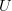$U$ of users, and a set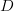$D$ of items. Let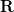$\mathbf{R}$ of size$|U| \times |D|$ be the matrix that contains all the ratings that the users have assigned to the items. Also, we assume that we would like to discover$K$latent features. Our task, then, is to find two matrics matrices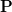$\mathbf{P}$ (a$|U| \times K$ matrix) and$\mathbf{Q}$ (a$|D| \times K$ matrix) such that their product approximates$\mathbf{R}$: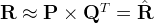$\mathbf{R} \approx \mathbf{P} \times \mathbf{Q}^T = \hat{\mathbf{R}}$ In this way, each row of$\mathbf{P}$ would represent the strength of the associations between a user and the features. Similarly, each row of$\mathbf{Q}$ would represent the strength of the associations between an item and the features. To get the prediction of a rating of an item$d_j$ by$u_i$, we can calculate the dot product of the two vectors corresponding to$u_i$ and$d_j$:$\hat{r}_{ij} = p_i^T q_j = \sum_{k=1}^k{p_{ik}q_{kj}}$ Now, we have to find a way to obtain$\mathbf{P}$ and$\mathbf{Q}$. One way to approach this problem is the first intialize the two matrices with some values, calculate how different’ their product is to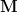$\mathbf{M}$, and then try to minimize this difference iteratively. Such a method is called gradient descent, aiming at finding a local minimum of the difference. The difference here, usually called the error between the estimated rating and the real rating, can be calculated by the following equation for each user-item pair:$e_{ij}^2 = (r_{ij} - \hat{r}_{ij})^2 = (r_{ij} - \sum_{k=1}^K{p_{ik}q_{kj}})^2$ Here we consider the squared error because the estimated rating can be either higher or lower than the real rating. To minimize the error, we have to know in which direction we have to modify the values of$p_{ik}$ and$q_{kj}$. In other words, we need to know the gradient at the current values, and therefore we differentiate the above equation with respect to these two variables separately:$\frac{\partial}{\partial p_{ik}}e_{ij}^2 = -2(r_{ij} - \hat{r}_{ij})(q_{kj}) = -2 e_{ij} q_{kj}$$\frac{\partial}{\partial q_{ik}}e_{ij}^2 = -2(r_{ij} - \hat{r}_{ij})(p_{ik}) = -2 e_{ij} p_{ik}$ Having obtained the gradient, we can now formulate the update rules for both$p_{ik}$ and$q_{kj}$: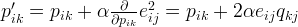$p'_{ik} = p_{ik} + \alpha \frac{\partial}{\partial p_{ik}}e_{ij}^2 = p_{ik} + 2\alpha e_{ij} q_{kj}$$q'_{kj} = q_{kj} + \alpha \frac{\partial}{\partial q_{kj}}e_{ij}^2 = q_{kj} + 2\alpha e_{ij} p_{ik}$ Here,$\alpha$ is a constant whose value determines the rate of approaching the minimum. Usually we will choose a small value for$\alpha$, say 0.0002. This is because if we make too large a step towards the minimum we may run into the risk of missing the minimum and end up oscillating around the minimum. A question might have come to your mind by now: if we find two matrices$\mathbf{P}$ and$\mathbf{Q}$ such that$\mathbf{P} \times \mathbf{Q}$ approximates$\mathbf{R}$, isn’t that our predictions of all the unseen ratings will all be zeros? In fact, we are not really trying to come up with$\mathbf{P}$ and$\mathbf{Q}$ such that we can reproduce$\mathbf{R}$ exactly. Instead, we will only try to minimise the errors of the observed user-item pairs. In other words, if we let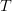$T$ be a set of tuples, each of which is in the form of$(u_i, d_j, r_{ij})$, such that$T$ contains all the observed user-item pairs together with the associated ratings, we are only trying to minimise every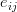$e_{ij}$ for$(u_i, d_j, r_{ij}) \in T$. (In other words,$T$ is our set of training data.) As for the rest of the unknowns, we will be able to determine their values once the associations between the users, items and features have been learnt. Using the above update rules, we can then iteratively perform the operation until the error converges to its minimum. We can check the overall error as calculated using the following equation and determine when we should stop the process.$E = \sum_{(u_i,d_j,r_{ij}) \in T}{e_{ij}} = \sum_{(u_i,d_j,r_{ij}) \in T}{(r_{ij} - \sum_{k=1}^K{p_{ik}q_{kj}})^2}$ # Regularization The above algorithm is a very basic algorithm for factorizing a matrix. There are a lot of methods to make things look more complicated. A common extension to this basic algorithm is to introduce regularization to avoid overfitting. This is done by adding a parameter$\beta$ and modify the squared error as follows:$e_{ij}^2 = (r_{ij} - \sum_{k=1}^K{p_{ik}q_{kj}})^2 + \frac{\beta}{2} \sum_{k=1}^K{(||P||^2 + ||Q||^2)}$ In other words, the new parameter$\beta$ is used to control the magnitudes of the user-feature and item-feature vectors such that$P$ and$Q$ would give a good approximation of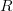$R$ without having to contain large numbers. In practice,$\beta$ is set to some values in the range of 0.02. The new update rules for this squared error can be obtained by a procedure similar to the one described above. The new update rules are as follows.$p'_{ik} = p_{ik} + \alpha \frac{\partial}{\partial p_{ik}}e_{ij}^2 = p_{ik} + \alpha(2 e_{ij} q_{kj} - \beta p_{ik} )$$q'_{kj} = q_{kj} + \alpha \frac{\partial}{\partial q_{kj}}e_{ij}^2 = q_{kj} + \alpha(2 e_{ij} p_{ik} - \beta q_{kj} )$ # Implementation in Python Once we have derived the update rules as described above, it actually becomes very straightforward to implement the algorithm. The following is a function that implements the algorithm in Python (note that this implementation requires the numpy module).  01 import numpy  02  03 def matrix_factorization(R, P, Q, K, steps=5000, alpha=0.0002, beta=0.02):  04  Q = Q.T  05  for step in xrange(steps):  06  for i in xrange(len(R)):  07  for j in xrange(len(R[i])):  08  if R[i][j] > 0:  09  eij = R[i][j] - numpy.dot(P[i,:],Q[:,j])  10  for k in xrange(K):  11  P[i][k] = P[i][k] + alpha * (2 * eij * Q[k][j] - beta * P[i][k])  12  Q[k][j] = Q[k][j] + alpha * (2 * eij * P[i][k] - beta * Q[k][j])  13  eR = numpy.dot(P,Q)  14  e = 0  15  for i in xrange(len(R)):  16  for j in xrange(len(R[i])):  17  if R[i][j] > 0:  18  e = e + pow(R[i][j] - numpy.dot(P[i,:],Q[:,j]), 2)  19  for k in xrange(K):  20  e = e + (beta/2) * (pow(P[i][k],2) + pow(Q[k][j],2))  21  if e < 0.001:  22  break  23  return P, Q.T We can try to apply it to our example mentioned above and see what we would get. Below is a code snippet in Python for running the example.  01 R = [  02  [5,3,0,1],  03  [4,0,0,1],  04  [1,1,0,5],  05  [1,0,0,4],  06  [0,1,5,4],  07  ]  08  09 R = numpy.array(R)  10  11 N = len(R)  12 M = len(R)  13 K = 2  14  15 P = numpy.random.rand(N,K)  16 Q = numpy.random.rand(M,K)  17  18 nP, nQ = matrix_factorization(R, P, Q, K)  19 nR = numpy.dot(nP, nQ.T) And the matrix obtained from the above process would look something like this:  D1 D2 D3 D4 U1 4.97 2.98 2.18 0.98 U2 3.97 2.40 1.97 0.99 U3 1.02 0.93 5.32 4.93 U4 1.00 0.85 4.59 3.93 U5 1.36 1.07 4.89 4.12 We can see that for existing ratings we have the approximations very close to the true values, and we also get some ‘predictions’ of the unknown values. In this simple example, we can easily see that U1 and U2 have similar taste and they both rated D1 and D2 high, while the rest of the users preferred D3, D4 and D5. When the number of features (K in the Python code) is 2, the algorithm is able to associate the users and items to two different features, and the predictions also follow these associations. For example, we can see that the predicted rating of U4 on D3 is 4.59, because U4 and U5 both rated D4 high. # Further Information We have discussed the intuitive meaning of the technique of matrix factorization and its use in collaborative filtering. In fact, there are many different extensions to the above technique. An important extension is the requirement that all the elements of the factor matrices ($\mathbf{P}$ and$\mathbf{Q}$ in the above example) should be non-negative. In this case it is called non-negative matrix factorization (NMF). One advantage of NMF is that it results in intuitive meanings of the resultant matrices. Since no elements are negative, the process of multiplying the resultant matrices to get back the original matrix would not involve subtraction, and can be considered as a process of generating the original data by linear combinations of the latent features. # Factorization Machines A Theoretical Introduction ## Factorization Machines This post is going to focus on explaining what factorization machines are and why they are important. The future posts will provide practical modeling examples and a numpy clone implementation of factorization machines. ### What problem do Factorization Machines solve? TL;DR: FMs are a combination of linear regression and matrix factorization that models sparse feature interactions but in linear time. Normally when we think of linear regression, we think of this formula.In the formula above, the run time is O(n) where n is the number of features. When we consider quadratic feature interactions, the complexity increases to O(n^2), in the formula below.Now consider a very sparse set of features, the runtime blows up. In most cases, we instead model a very limited set of feature interactions to manage the complexity. ### How do Factorization Machines solve the problem? In the recommendation problem space, we have historically dealt with the sparsity problem with a well documented technique called (non-negative) matrix factorization.We factorize sparse user item matrix (r) R^{UxI} into a user matrix (u) R^{UxK} and an item matrix (i) ^R^{IxK}, where K<<U and K<<I . User (u_i)’s preference for item i_j can be approximated by u_ii_j Factorization Machines takes inspiration from matrix factorization, and models the feature iteractions like using latent vectors of size K. As a result, every sparse feature f_i has a corresponding latent vector v_i. And two feature’s interactions are modelled as v_iv_j ### Factorization Machines MathInstead of modeling feature interactions explicitly, factorization machines uses the dot product of features’ interactions. The model learns v_i implicitly during training via techniques like gradient descent. The intuition is that each feature will learn a dense encoding, with the property that high positive correlation between two features have a high dot product value and vise versa. Of course, the latent vectors can only encode so much. There is an expected hit on accuracy compared to using conventional quadratic interactions. The benefit is that this model will run in linear time. ### FM complexity For the full proof, see lemma 3.1 in Rendle’s paper.Sincecan be precomputed, the complexity is reduced to O(KN). # Installing Apache Spark on Ubuntu 16.04 The following installation steps worked for me on Ubuntu 16.04. The below options worked for me: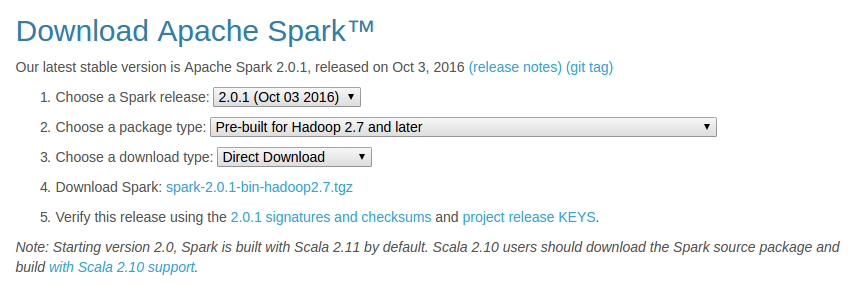Download Apache Spark • Unzip and move Spark cd ~/Downloads/ tar xzvf spark-2.0.1-bin-hadoop2.7.tgz mv spark-2.0.1-bin-hadoop2.7/ spark sudo mv spark/ /usr/lib/  • Install SBT As mentioned at sbt – Download echo "deb https://dl.bintray.com/sbt/debian /" | sudo tee -a /etc/apt/sources.list.d/sbt.list sudo apt-key adv --keyserver hkp://keyserver.ubuntu.com:80 --recv 2EE0EA64E40A89B84B2DF73499E82A75642AC823 sudo apt-get update sudo apt-get install sbt  • Make sure Java is installed If not, install java sudo apt-add-repository ppa:webupd8team/java sudo apt-get update sudo apt-get install oracle-java8-installer  • Configure Spark cd /usr/lib/spark/conf/ cp spark-env.sh.template spark-env.sh vi spark-env.sh  Add the following lines JAVA_HOME=/usr/lib/jvm/java-8-oracle SPARK_WORKER_MEMORY=4g  • Configure IPv6 Basically, disable IPv6 using sudo vi /etc/sysctl.conf and add below lines net.ipv6.conf.all.disable_ipv6 = 1 net.ipv6.conf.default.disable_ipv6 = 1 net.ipv6.conf.lo.disable_ipv6 = 1  • Configure .bashrc I modified .bashrc in Sublime Text using subl ~/.bashrc and added the following lines export JAVA_HOME=/usr/lib/jvm/java-8-oracle export SBT_HOME=/usr/share/sbt-launcher-packaging/bin/sbt-launch.jar export SPARK_HOME=/usr/lib/spark export PATH=$PATH:$JAVA_HOME/bin export PATH=$PATH:$SBT_HOME/bin:$SPARK_HOME/bin:$SPARK_HOME/sbin  • Configure fish (Optional – But I love the fish shell) Modify config.fish using subl ~/.config/fish/config.fish and add the following lines #Credit: http://fishshell.com/docs/current/tutorial.html#tut_startup set -x PATH$PATH /usr/lib/spark
set -x PATH $PATH /usr/lib/spark/bin set -x PATH$PATH /usr/lib/spark/sbin

• Test Spark (Should work both in fish and bash)

Run pyspark (this is available in /usr/lib/spark/bin/) and test out.

For example ….

>>> a = 5
>>> b = 3
>>> a+b
8
>>> print(“Welcome to Spark”)
Welcome to Spark
## type Ctrl-d to exit


Try also, the built in run-example using run-example org.apache.spark.examples.SparkPi`

That’s it! You are ready to rock on using Apache Spark!